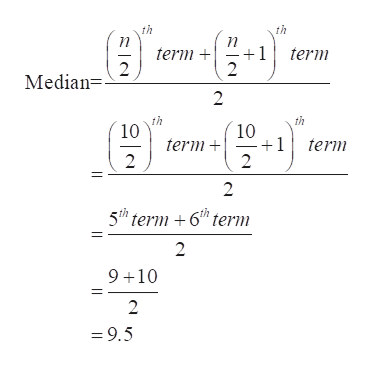# Using the data below (assume the data comes from a population)5,8,14,6,21,11,9,10,18,2A.  Calculate the three measures of central tendencyB. Calculate the standard deviation of the data and what the variance isC. Draw a box plotD. Using the IQR X 1.5(Interquartile Range), Can you identify any outliers in the data?

Question
45 views

Using the data below (assume the data comes from a population)

5,8,14,6,21,11,9,10,18,2

A.  Calculate the three measures of central tendency

B. Calculate the standard deviation of the data and what the variance is

C. Draw a box plot

D. Using the IQR X 1.5(Interquartile Range), Can you identify any outliers in the data?

check_circle

Step 1

Hello. Since your question has multiple sub-parts, we will solve first three sub-parts for you. If you want remaining sub-parts to be solved, then please resubmit the whole question and specify those sub-parts you want us to solve.

Step 2

Consider, X = 5, 8, 14, 6, 21, 11, 9, 10, 18, 2.

(a)

Three measures of central tendency are mean, median and mode.

Here, mean using the provided data can be calculated as:

Step 3

Median is the middle most value of the arranged data set, which divides the data into two equal parts.

In order to calculate median, arrange the provided data in ascen...help_outlineImage Transcriptioncloseth th n 1 2 term 2 term Median= 2 th 10 th 10 +1 2 term term 2 2 5th term 6 term 2 9 10 2 = 9.5 fullscreen

### Want to see the full answer?

See Solution

#### Want to see this answer and more?

Solutions are written by subject experts who are available 24/7. Questions are typically answered within 1 hour.*

See Solution
*Response times may vary by subject and question.
Tagged in

### Data